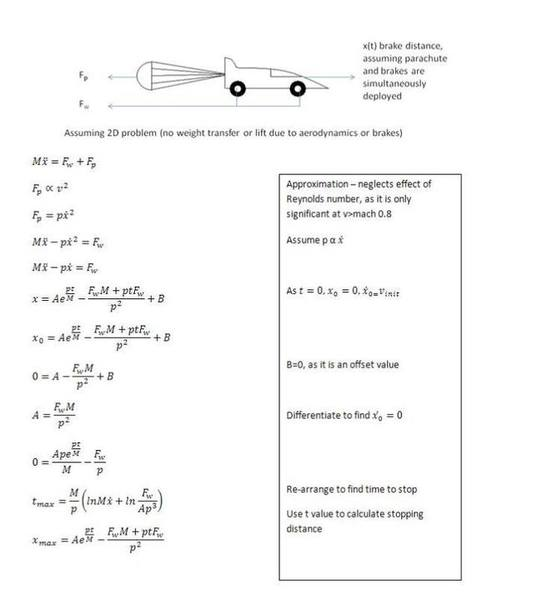Formula for deceleration, using a parachute and wheel brakes

Homework Statement

Basically i need to find the braking distance and time for the deceleration of a vehicle for the following conditions,

using a parachute
using a parachute, and wheel brakes

does anyone know of a suitable formula to calculate this?

Homework Equations

Fd=0.5CdPAu^2 - drag force from parachute
Fw= Constant - brake force at wheels - Ive already calculated this to be in the region of 7000N - though this number is likely to change

The Attempt at a Solution

Basically i have the following differential equation, which im not sure if it is correct, it has also been a very long time since i solved a differential equation:
M d2x/dt^2 + P dx/dt + B = 0 where M is the mass, Fd is the drag force from the parachute and Fw is constant force (brakes.)

if this is correct, how might i go about calculating a stopping distance, and time to stop?

Many Thanks

Ed

Last edited:

distance,
s=(v²-u²)/2a
where:
s is the displacement
v is the end velocity (0 for when vehicle is stopped)
u is inital velocity
a is acceleration (will be a negative number for deceleration)

You get your a from F=ma where m is the mass of the vehicle and F is your total stopping force (will be negative to give a deceleration)

be careful, the kinematics equation for distance is only applicable for constant deceleration, but your force generated by your parachute will decrease as your velocity decreases and so your deceleration will decrease. You will probably assume that your wheel braking force is constant throughout?

for changing accelerations, you'll could work out you acceleration due to the parachute over a few velocities to find a solution for, say, every 1meter, or you'll have to use some integration.

I'm not sure your drag force equation is correct, where did you get that from? usually drag is in the form Fd=0.5*density*frontal area*velocity^2

your correct with the drag force - typo on my part - i accidentally did the power formula... edited now anyway..

The formulae ive been using for stopping distances and time for the wheel brakes alone is as follows

X=(V^2M)/(2Fw)
t=VM/Fw

The newton equations do work for constant brake forces as stated, but i will need to use a differential equation to calculate the variable braking force.

Cheers
Ed

PhanthomJay
Homework Helper
Gold Member
I don't quite follow your equation; The forces acting on the train are the braking force , F_w, and the quadratic drag force, F_d. Newton's 2nd law then gives the equation
Mdv/dt(or Md^2x/dt^2) = F_d + F_w, where F_d is the appropriate quadratic drag (as a function of the velocity, v), and F_w is the constant braking force. The solution of this differential equation, once you have the correct drag term, is a bit messy; you might get some help here
www.brightlemon.com/ma/what_use/TractiveEffortAccelerationAndBraking.doc[/URL]

Last edited by a moderator:
Hi All,

Thanks for all your help so far, the following is where i have got to working with it - would someone be able to check my derivation of this please - my maths skills are somewhat rusty, so i may well have made a mistake somewhere.Cheers
Ed